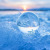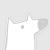IEEE控制论会刊的范围包括控制论领域的计算方法。该期刊欢迎关于跨机器或机器、人和组织之间的通信和控制的论文。其范围包括计算智能、计算机视觉、神经网络、遗传算法、机器学习、模糊系统、认知系统、决策制定和机器人技术等领域，只要这些领域对控制论的主题有所贡献或展示了控制论原理的应用。 官网地址：http://dblp.uni-trier.de/db/journals/tcyb/0+0+0+0+0+0+0+0+0+0+0+0+0+0+0+0+0+0+0+0+0+0+0+0+0+1+0+0+4+0+0+0+0+0+0+0+0+0+0+0+1+0+0+3+0+
Top